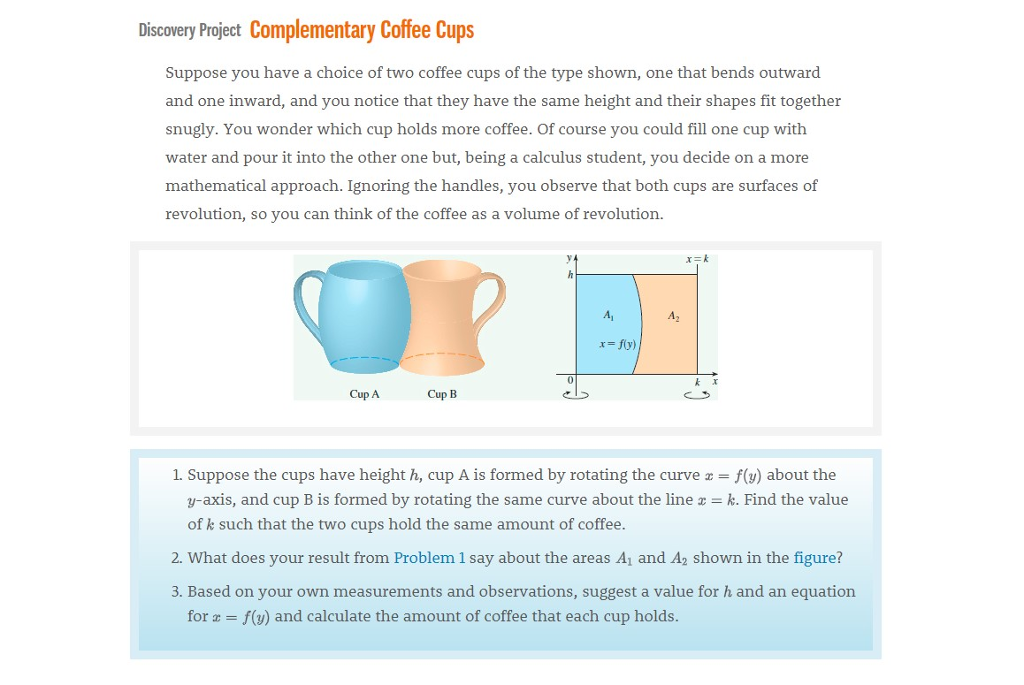# Sec.8.3 - Complementary Coffee Cups

Applied Project in Sec.8.3, Calculus by Stewart Chinese version: Complementary Coffee Cups

Suppose you have a choice of two coffee cups of the type shown, one that bends outward and one inward, and you notice that they have the same height and their shapes fit together snugly. You wonder which cup holds more coffee. Of corse you could fill one cup with water and pour it into the other one but, being a calculus student, you decide on a more mathematical approach. Ignoring the handles, you observe that both cups are surfaces of revolution, so you can think of the coffee as a volume of revolution.Question 1:

Suppose the cups have height $h$, cup A is formed by rotating the curve $x=f(y)$ about the $y$-axis, and cup B is formed by rotating the same curve about the $x=k$. Find the value of $k$ such that two cups hold the same amount of coffee.

$$k=\frac{2\int_0^{h}f(y)dy}{h}.$$

Question 2:

What does your result from Problem 1 say about the areas $A_1$ and $A_2$ shown in the figure?

when Cup A’s volume equals Cup B’s, the area of $A_1$ is the same as $A_2$.

Question 3:

Use Pappus’s Theorem to explain your result in Problem 1 and 2.

Pappus’s Theorem

Let $R$ be a plane region that lies entirely on one side of a line $l$ in the plane. If $R$ is rotated about $l$, then the volume of the resulting solid is the product of the area $A$ of $R$ and the distance $d$ travled by the centroid of $R$.